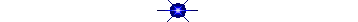MathFourth Grade instructional time will focus on three critical areas:

(1) developing an understanding of and fluency with multi-digit multiplication, and developing an understanding of division to find and interpret quotients involving multi-digit dividends;

(2) developing an understanding of fraction equivalence, addition and subtraction of fractions with like denominators, and multiplication of fractions by whole numbers;

(3) understanding that geometric figures can be analyzed and classified based on their properties, such as having parallel sides, perpendicular sides, particular angle measures, and symmetry.# Mathematical Practices

Make sense of problems and persevere in solving them.

Keep trying!

I solve problems without giving up.

Reason abstractly and quantitatively.

I think about numbers in many ways.

Construct viable arguments and critique the reasoning of others.

I explain my thinking and try to understand.

Model with mathematics.

Model math!

I show my work in a variety of ways.

Use appropriate tools strategically.

Use math tools!

I use math tools and tell why I chose them.

Attend to precision.

I work carefully and check my work for accuracy.

Look for and make use of structure.

Look for patterns!

I use what I know to solve new problems.

Look for and express regularity in repeated reasoning.

Look for shortcuts!

I solve problems by looking for rules and patterns.Numbers & Operations in Base Ten ·    Generalize place value understanding for multi-digit numbers. ·    Use place value understanding and properties of operations to perform       multi-digit arithmetic. Number Monster Rounding Off Hangman Rounding One False Move Mystery Picture Rounding Flashcards Place Value Hockey Place Value Millionaire Mystery NumbersOperations & Algebraic Thinking ·  Use the four operations with whole numbers to solve problems. ·  Gain familiarity with factors and multiples. ·  Generate and analyze patterns. IXL All skills organized within Common Core Stanards. Mr. Nussbaum’s Math Lab Power Football Interactive Multiplication Grid Math Mayhem Operation Order Bracket Basics Math Cats Factors & Multiples Multiplication Arrays Thinking Blocks Make ArraysNumbers & Operations - Fractions ·  Extend understanding of fraction equivalence and ordering. ·  Build fractions from unit fractions by applying and extending previous      understandings of operations on whole numbers. ·  Understand decimal notation for fractions and compare decimal            fractions. Fraction Puzzle Mixed # to Improper Fraction Fraction Games! Intergalactic Space Games Pizza Fractions Equivalent Fraction Match Fraction of a Set Simplify Fractions Fraction Frenzy Ordering Fractions Death to Decimals EZ Fractions DecimalsMeasurement & Data ·    Solve problems involving measurement and conversion of measurements       from a larger unit to a smaller unit. ·    Represent and interpret data. ·    Geometric measurement: understand concepts of angles and measuring     angles. Soda Jerk Pour to Score Isometric Drawing Measure It Area + Perimeter Fill the Box The Data Bank Create a Graph Elapsed Time Bang on Time Alien Angles Measuring AnglesGeometry ·         Draw and identify lines and angles. ·         Classify shapes by properties of their lines and angles. Angle Definitions Line Game Quadrilateral Game Geometric Figures Congruent Games Angle Sort 1 Angle Sort 2 Hit the Target Fruit Picker Angles Stargazing Mirror Game Symmetry Game Solids and Nets